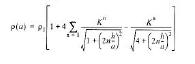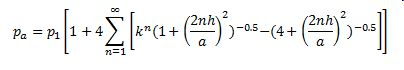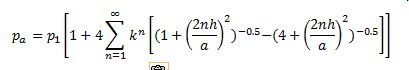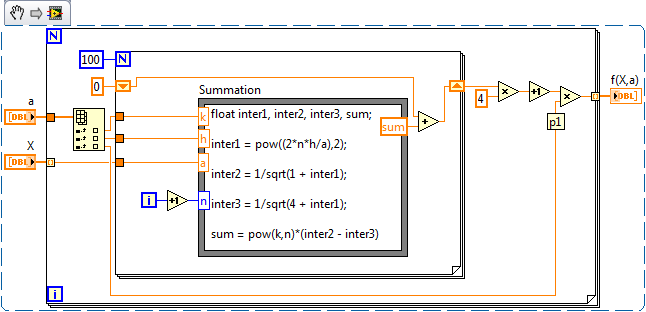# LabVIEW

cancel
Showing results for
Did you mean:

## Fitting an Optimal Curve and Finding Variables

Hi all,

I have measured data and a (non-linear?) function which describes the data.I want to fit a curve to the measured data to determine an optimal set of values for the independent variables K, h and p1.

I have been given a solution which uses gradient descent method (i.e. partial derivatives) and aims at minimising some gradient error function.

I was thinking that I could do this much more easily using perhaps the Levenberg-Marquardt VI which I have read a bit about in the forums.

I have no idea how to tackle this problem with the equation that I'm using.

I hope that somebody can help me.

Much appreciated.

Battler.

Message 1 of 23
(3,124 Views)

## 回复： Fitting an Optimal Curve and Finding Variables

Hi Battler,

Could you please re-post your equation?   The equation figure is difficult to identify.

You might want to start from the nonlinear curve fit VI examples which listed in Nonlinear Curve Fit VI help.

Best Regards,

Michael

Message 2 of 23
(3,112 Views)

## Re: 回复： Fitting an Optimal Curve and Finding Variables

Hi Michael,I am currently looking at the non-linear curve fit.  I do not understand the dimensions of my problem and how to go about it.

I even dont really understand the basic principles.

Help is Greatly appreciated.

Thanks.

Message 3 of 23
(3,103 Views)

## Re: 回复： Fitting an Optimal Curve and Finding VariablesThe previous equation wasn't quite correct.

How to go about it and the basic principles of non-linear curve fitting.

What are the dimensions of the problem?  Is it greater than 1D or 2D?  I need to derive p1, k and h.

As you can see my equation is an infinite sum.  Is this ok?

Thanks again.

Message 4 of 23
(3,095 Views)

## Re: Fitting an Optimal Curve and Finding Variables

An example of actual measured data is:

a =          pa =

2.5          320

5             245

7.5          182

10           162

12.5        168

15           152

Message 5 of 23
(3,084 Views)

## Re: Fitting an Optimal Curve and Finding Variables

Have you looked at the non-linear curve fitting examples shipped with LV?

They're a pretty good starting point.  All you need is to develop a VI which takes an array of your variables and calculates the result.  The Lev-Mar framework does the rest for you.

Shane.

Message 6 of 23
(3,078 Views)

## Re: Fitting an Optimal Curve and Finding Variables

I have looked at the shipped example.

Do I need to create a VI which contains my equation?  It contains an infinite summation.

How do I go about building the equation?  Will I pass a 2D array??

Message 7 of 23
(3,064 Views)

## Re: Fitting an Optimal Curve and Finding Variables

If you are unable to express your equation, then it was perhaps a bit premature of you to post a question on non-linear optimisation of said equation I think.

Shane.

Message 8 of 23
(3,057 Views)

## Re: Fitting an Optimal Curve and Finding VariablesIs that correct?  I've gone with a summation of 100 iterations.  Could I use a while loop?

Message 9 of 23
(3,049 Views)

## Re: Fitting an Optimal Curve and Finding Variables

As battler. is about to figure out for himself, I am not a big fan of estimating infinite series by first N terms, I prefer to wait for two consecutive "small" terms.  He will most likely choose to wait for a single small term, but some series can trick you when either the even or odd terms are always small.  Here is an example.  The steps are the same as any other non-linear fit, just a little more effort to find f(x,a).  I'll let the partial derivatives slide in this case.Message Edited by Darin.K on 02-12-2010 03:04 PM
Message 10 of 23
(3,040 Views)